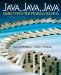# Chapter Summary

[Page 459 (continued)]

#### Technical Terms

array

array initializer

array length

binary search

data structure

element

element type

insertion sort

multidimensional array

one-dimensional array

polymorphic sort method

selection sort

sequential search

sorting

subscript

two-dimensional array

#### Summary of Important Points

• An array is a named collection of contiguous storage locations, each of which stores a data item of the same data type. Each element of an array is referred to by a subscriptthat is, by its position in the array. If the array contains N elements, then its length is N and its indexes are 0, 1, . . . N-1.

• [Page 460]
• Array elements are referred to using the following subscript notation arrayname[subscript], where arrayname is any valid identifier, and subscript is an integer value in the range 0 to arrayname.length - 1. The array's length instance variable can be used as a bound for loops that process the array.

• An array declaration provides the name and type of the array. An array instantiation uses the keyword new and causes the compiler to allocate memory for the array's elements:

`int arr[];          // Declare a one-dimensional array variable arr = new int;  // Allocate 15 int locations for it `

• Multidimensional arrays have arrays as their components:

`int twoDarr[][];            // Declare a two-dimensional array variable twoDarr = new int;  // Allocate 150 int locations `

• An array's values must be initialized by assigning values to each array location. An initializer expression may be included as part of the array declaration.

• Insertion sort and selection sort are examples of array-sorting algorithms. Both algorithms require several passes over the array.

• When an array is passed as an argument to a method, a reference to the array is passed rather than the entire array.

• Swapping two elements of an array, or any two locations in memory, requires the use of a temporary variable.

• Sequential search and binary search are examples of array-searching algorithms. Binary search requires that the array be sorted.

• For multidimensional arrays, each dimension of the array has its own length variable.

• Inheritance and polymorphism are useful design features for developing a hierarchy of computer games.Java, Java, Java, Object-Oriented Problem Solving (3rd Edition)
ISBN: 0131474340
EAN: 2147483647
Year: 2005
Pages: 275

Similar book on Amazon